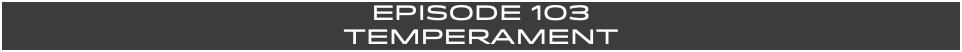HOSTS- Jeremy Burns, Matthew Scott Phillips

TYPE- Special Topics

DURATION- 78:12

BUMPER MUSIC- "Bad Temperament" (Area 47)

ANNOUNCER- Mike Cunliffe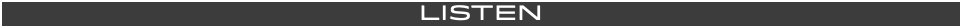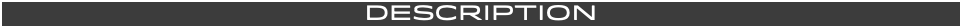Today's most widely used tuning system is equal temperament. It sounds great to our ears. But it is a compromise! Musicians and mathematicians have always struggled finding tuning systems that didn't compromise the pure tones provided within the natural harmonic series. On this episode, we will discuss these struggles and the systems that arose from this effort: just tuning, Pythagorean tuning, meantone tuning, well temperament and equal temperament!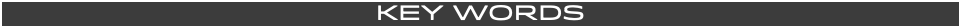TONE- A musical sound that rings at a certain frequency. The frequency determines what pitch the tone will sound as.

FREQUENCY- The number of vibrations per second. Frequencies are measured in hertz (Hz). For example, the note A 440Hz vibrates at a frequency of 440 time per second.

HERTZ (Hz)- The unit of measurement that is determined by the amount of times a moving body vibrates per second.

CENT- A unit of measurement that the divides the semitone. 1 semitone= 100 cents.

FUNDAMENTAL- This is the most prominent tone that rings out. The tone that rings as the fundamental is often the note that is played. For example, if you play the A note above middle C, than that note (A 440 Hz) is considered the fundamental. This is usually the most audible tone.

OVERTONE- This is a frequency or tone that is added to another tone. This is also referred to as a "harmonic". The FUNDAMENTAL is considered the "1st harmonic". The "2nd harmonic" is known as the "1st overtone".

TEMPERAMENT- How a scale is tuned, or how the intervals between notes are spaced.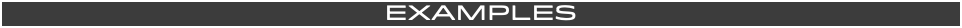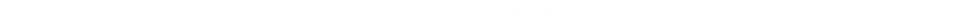When something is tuned JUST or PURE, it relies on intervals based on simple ratios. The main focal points of this system were the OCTAVES (P8) and the 5THS (P5). Here are the simple ratios for these harmonics, using A 220:

FUNDAMENTAL- 220 Hz (unison)= 1:1 (fundamental x 1)

OCTAVE 440 Hz (1 Octave)= 2:1 (fundamental x2)

PURE 5th- 330 Hz (P5)= 3:2 ( fundamental x 1.5)

The latter ratio, 3:2, was held sacred by Pythagoras (c. 570 – c. 495 BCE). This mathematician found that after plucking a string (for example one tuned at A 220), plucking one 1/2 the length (2:1 at 440Hz) in made a very pleasing harmony. Next pleasing was a string a 2/3 the length (3:2). These are the first harmonics of the overtone series. This priority of pure tones was the basis of the PYTHAGOREAN TUNING SYSTEM.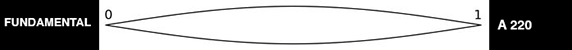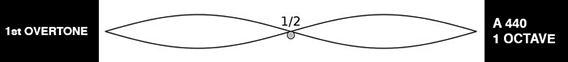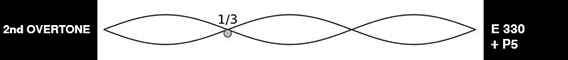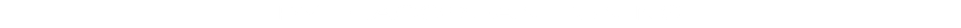This tuning system, developed by Pythagoras (6th century B.C.E.). It prioritized on the PURE or JUST 5th (P5), as was the music of the centuries that followed up until the Renaissance period (1500's C.E.).

In order to have more pure 5ths, within the octave, the purity of the 3rds and 6ths had to be compromised. The 5ths were built by multiplying the fundamental by 1.5.

In this system the, sharp notes (major intervals) were sharper by a few cents and the flat notes (minor intervals) were flatted by a few cents. But the 5th were absolutely perfect.

The PYTHAGOREAN COMMA- If we keep on using the 3:2 ratio to move up a pure or just 5th, by the time we get back to the root after moving up the circle of 5th’s we run into a problem. Let’s listen!

If we start at A (220Hz) and want to find the OCTAVE, we multiply it by 2. We get A (440Hz).

The relationship between 440 and 220 are considered to that of a 2:1 ratio.

If we start at A (220Hz) and want to find the PURE 5TH, we multiply it by 1.5. We get E (330Hz).

The relationship between 330 and 220 are considered to that of a 3:2 ratio. However, the corresponding E note on a modern day EQUALLY TEMPERED piano is tuned to 329.268Hz. This is a difference of

.732 Hz.

If we want to stack another PURE 5TH on top of that 330, we multiply by 1.5 to arrive at a B (495Hz). However, the corresponding B note on a modern day EQUALLY TEMPERED piano is tuned to 493.8Hz. This is a difference of 1.2 Hz. The distance has widened between the PURE 5TH and the EQUALLY TEMPERED 5TH in the previous harmonic.

By the time this pattern of stacking PURE 5THS returns to the A note, we get A (28544.1936Hz). In order to find a sensible frequency to compare, we divide this ultra high number by 32 to land on 892 Hz. But if we keep doubling the octaves, we should have landed at 880 Hz. This is a difference of 12 Hz!

This 12 Hz discrepancy, based on the fundamental of A, is known to be the PYTHAGOREAN COMMA. What ever fundamental you begin with, this method of stacking PURE 5THS will result in a COMMA (whatever the size).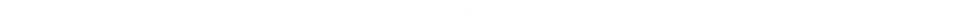In the Renaissance period, composers like John Dunstable began adding the mediant harmonies of the 3rds and 6ths to their compositions, giving these intervals more popularity.

For vocalists, singing pure 3rds and 6th was attainable. But now, the previous system had to "TEMPERED" with in order to play the pure 3rds and 6ths on accompanying instruments. And to do this, they had to adjust that once PURE 5th to accommodate for the now PURE 3rds and 6ths.

In the 1/4 MEANTONE SYSTEM, the 5th were flatted by a 1/4 of the COMMA.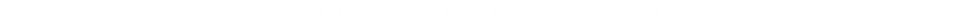The WELL TEMPERED TUNING system was closer to what we know of today. The semitones were not evenly divided but it allowed for playing in multiple keys, major and minor.

Among the more popular of those was Francesco Valotti’s system which was closer to MEANTONE for the first 6 notes and PYTHAGOREAN for the following 6. So the C major chord, for example would sound exquisite, on a C instrument. But the further away we get from that fundamental, the less perfect the harmonies become. So that B major, would be a more tense version of a major chord, in comparison to the C major chord.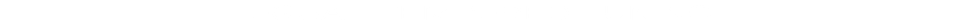In EQUAL TEMPERAMENT, the OCTAVE is divided into 12 equal semitones (1/2 steps).

Each 5th is smaller than the pure 5th by a 1/12 of the COMMA.

In this system, the 5ths are a little flat. The MAJOR 3rd is slightly sharp. The MINOR 3rd is slightly flat. But the discrepancies between these notes and their JUST counterparts are negligible to the modern ear.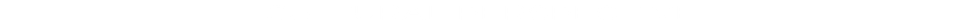In ancient China (circa 2679 B.C.E.), Ling Lun was credited for his design of the bamboo flutes which featured the five tones: Gong, Shang, Jiao, Chi, and Yu (scale steps 1, 2, 3, 5, 6 of the major pentatonic scale). Even today, their classical music is based on the just tuning system. Like Pythagoras and John Dunstaple, the Chinese culture believed in a deep connection with music in the cosmos. These pure intervals allow for a relaxing and fulfilling experience.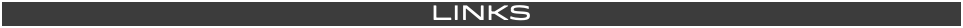by Leonard Bernstein

by Early Music Sources (Elam Rotem and Johannes Keller)

MUSIC STUDENT 101